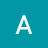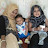Whenever, a quadratic equation cannot be factored, we can use the quadratic formula to get the solutions.

The standard form of a quadratic equation is ax^2+bx+c=0, where a, b, and c are real numbers. The value a should not be equal to 0.

There are 4 methods to solve a quadratic Equation.

1) Factoring method
3) Completing the square method
4) Graphing method

algebratutors.org

1.Abirami p says:
2.Fasila Pachu says: